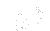This is an automatically generated documentation by LaTeX2HTML utility. In case of any issue, please, contact us at info@cfdsupport.com.

## How to model turbulence?

Turbulence modeling is extremely complicated task. The solution still arises from conservation laws. Governing equations are system of Navier-Stokes equations. The solution is always only some approximation. For description of turbulent flow, especially in technical applications, just the mean parameters of the fluid flow might be important.

There are two main approaches to numerical solution of governing equations. Either the mean fluid flow values are followed using an averaging approach – RANS. Or, the instantaneous fluid flow values are followed using direct approach – DNSLESDES.

In practice within real cases the most of solutions are based on Reynolds Averaged Navier-Stokes equations – RANS. RANS is based on decomposition23.3 of instantaneous values of variables into mean part and fluctuation part. Then the governing system called Reynolds Averaged Navier-Stokes equations (RANS) is solved for the mean values only and is completed by the turbulence model. Practically all the fluid flow is modeled (mean values) everywhere, no matter of time size or turbulent eddies scales.

The direct approach directly simulates all the instantaneous quantities and all the turbulence eddies scales in every time: Direct Numerical Simulation – (DNS). DNS requires very very fine mesh, especially near the walls. The number of vertices needed is proportional to, so the requirements quickly increase with increasing of Reynolds number. Currently23.4 DNS can be realized just on special high efficient computers only and nothing signalizes dramatic changes to come in near 23.5 future.

The compromise between DNS and RANS is Large Eddy Simulation (LES), or Detached Eddy Simulation (DES). Here the filter of eddies is applied. The large scale eddies are simulated directly but the small scale eddies are modeled applying the sub-grid model, typically some simple algebraic mixing-length model.

NOTE: The turbulence modeling approach always depends on how much detailed information (in space and time) is needed to meet the goals.

Subsections